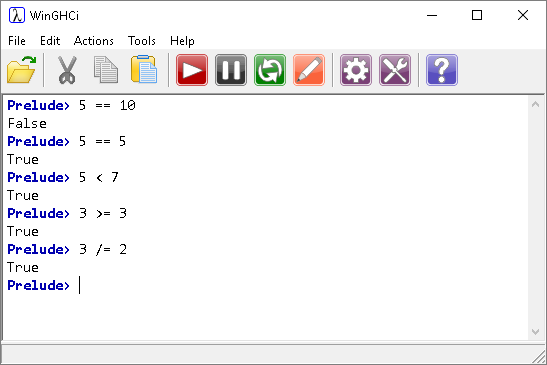# Introduction To Haskell Comparison Operators

## Introduction

We use comparison operators in expressions that evaluate to True or False. Apart from one little surprise with the not equal operator, these are also as you might expect.

 < Less than <= Less than or equal == Equal /= Not equal >= Greater than or equal > Greater than